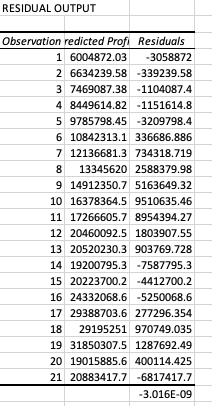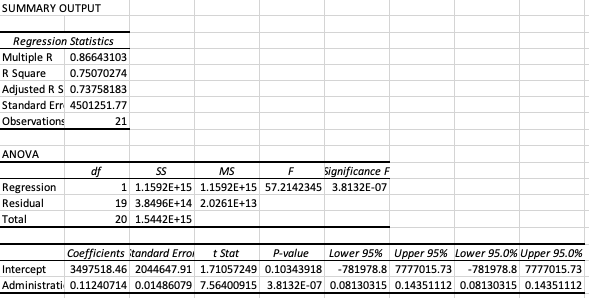Search
• Alice

# Optimisation and Forecasting using Multivariate and Regression AnalysisMultivariate analysis is a powerful statistical, econometric tool that can be used for forecasting and optimisation. The method can estimate the relationship between multiple dependent variables and multiple independent variables.

More specifically, it can show how the value of the dependent variable changes when the independent variable varies.

In this blog post we wanted to show a real case study with real numbers that we have found for Specsavers.

For our initial calculations we used administration costs cross referenced with sales over a 20 year period so a fair amount of data points could be found.

Along with a correlation shown on the generated line graph, the statistics summary provides important insight, as shown and detailed further below. These data points give inference into the strength of the relationship, the “goodness” of fit for the data falling on the regression line, in all giving an indicator of how statistically sound the calculation is.Multiple R is the correlation between actual and predicted values of the dependant variable. If R is close to 1 or -1 then there is a strong relation between the 2 variables.

R2 is the model’s accuracy in explaining the dependant variable. Anything above 0.60 is considered significant which in our case is 0.75.

The level of statistical significance is often expressed as a p-value between 0 and 1. The smaller the p-value, the stronger the evidence that you should reject the null hypothesis. In our example, p-value is 3.8132E-07 which is very close to zero.

The outcome, as expected shows that there is a significant, strong relationship between the two factors and have a dependant correlation, although the direction of which is a slightly more qualitative matter.

The ideal data for this type analysis would be direct information on the products and relative variables. For example, information on marketing costs over certain periods (e.g. seasons) and cross referencing that with the sales over that period, this would be regression analysis.

To make the results more accurate, multiple regression analysis can be used to involve alternate, perhaps macroeconomic factors that ultimately affect sales. In this instance, using GDP, as well as cost of marketing would provide a clearer picture as to what extent these factors have an effect on sales.

In multivariate analysis the steps are much alike, finding and analysing the original residuals and then simply adding the additional variable(s) in order to see the correlation as a whole with added macroeconomic insight.

These statistical measure can provide:

•A deeper understanding of the relationship between the cost of advertising and sales

•Understanding the effectiveness of new advertising techniques

•Understanding what impact each advertising type has on sales

•Ability to understand patterns and build on recommendations for the upcoming year to reduce costs while optimising sales

This is a very simple example of using data in order to make financial decisions. We are working with large enterprises where we use 20+ different parameters in order to make data centric financial decisions. Drop us an email to info@icangroup.co.uk if you want to find out more about how we are using data science and machine learning for better decision making and forecasting purposes.

You might also like this post;

Data driven financial decisions

https://www.icangroup.co.uk/post/data-centric-financial-decisions